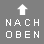### 100 Dollar Note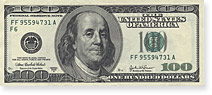W x H = 155.81 x 66.42 mm

### 50 Dollar Note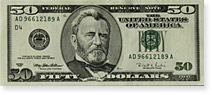W x H = 155.81 x 66.42 mm

### 20 Dollar Note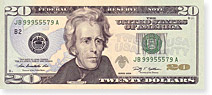W x H = 155.81 x 66.42 mm

### 10 Dollar Note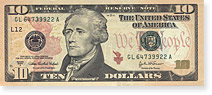W x H = 155.81 x 66.42 mm

### 5 Dollar Note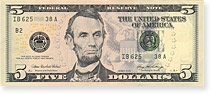W x H = 155.81 x 66.42 mm

### 2 Dollar Note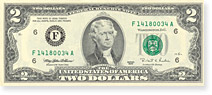W x H = 155.81 x 66.42 mm

### 1 Dollar Note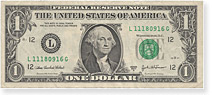W x H = 155.81 x 66.42 mm

# How much actually weigh ... 1 Million US Dollar?

If you have ever wondered how a 1 million Dollar looks like or how much a million 1 dollar bills weigh, you can calculate this simply below. I'm quite sorry. Unfortunately I just ran out of my millions otherwise I could have shown you how beautiful this can act on a photo. But I can tell you weight, height and volume of the money stack if you put everything neatly on each other ...

Dollar in
Every Dollar Note has the same weight - about 1 g and the size of 155.81 x 66.42 mm.
The amount of \$ 1,000,000 would have in 2 Dollar Notes a weight of 500.00 kg (1,102.31 pounds). A single stack of money with 500,000 new banknotes would be 50.00 m (54.68 yards) high und would have a volume of at least 517.45 litres (546.78 quarts).
For transportation, you should already have a vehicle.

Resource: Conversion of weights and measuresBitcoin calculator: Euro, Dollar and other currencies. How much does a Bitcoin cost? Convert Euros against Bitcoin and compare with other crypto currencies. Exchange rate calculator for 50 crypto and 32 fiat currencies.

## Currency Converter

And if you want to exchange your money the converter shows how much Swiss francs, Euros or British Pounds you will get for your Dollars.

### 1 Dollar Coin (Buck)Weight = 8.1 g,
Diameter = 26.5 mm
Thickness = 2 mm

### 50 Cent Coin (Half)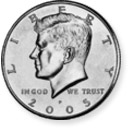Weight = 11.34 g,
Diameter = 30.61 mm
Thickness = 2.15 mm

### 25 Cent Coin (Quarter)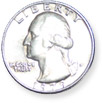Weight = 5.67 g,
Diameter = 24.26 mm
Thickness = 1.75 mm

### 10 Cent Coin (Dime)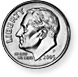Weight = 2.27 g,
Diameter = 17.91 mm
Thickness = 1.35 mm

### 5 Cent Coin (Nickel)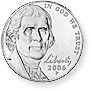Weight = 5 g,
Diameter = 21.21 mm
Thickness = 1.95 mm

### 1 Cent Coin (Penny)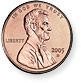Weight = 2.5 g,
Diameter = 19.05 mm
Thickness = 1.55 mm